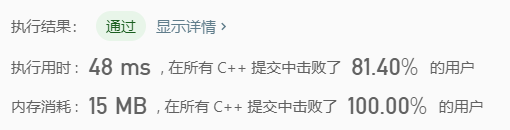# Leetcode实战： 392. 判断子序列

## 题目

s = "abc", t = "ahbgdc"



s = "axc", t = "ahbgdc"



## 算法实现：双指针

class Solution {
public:
bool isSubsequence(string s, string t) {
if (s=="") return 1;
int j(0), length(s.size());
for (int i = 0; i < t.size(); i++) {
if (t[i] == s[j]) j++;
if (j == length) return 1;
}
return 0;
}
};


## 结果©️2019 CSDN 皮肤主题: 大白 设计师: CSDN官方博客